# short long Division space saver Short division 369

• Slides: 14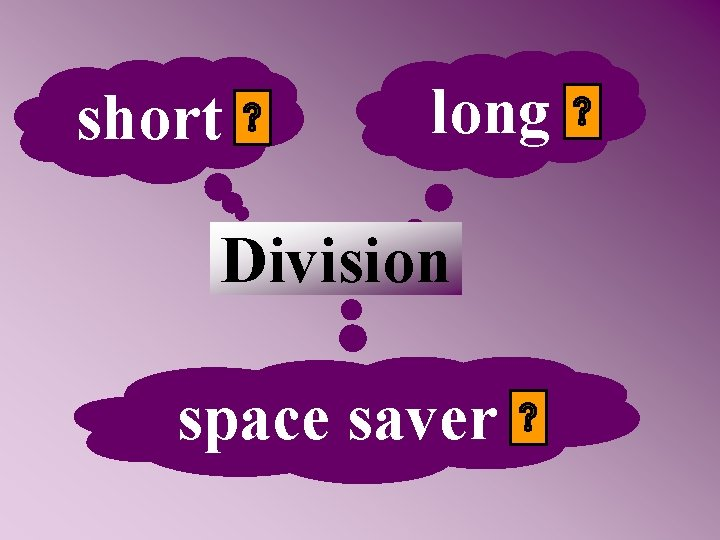short long Division space saver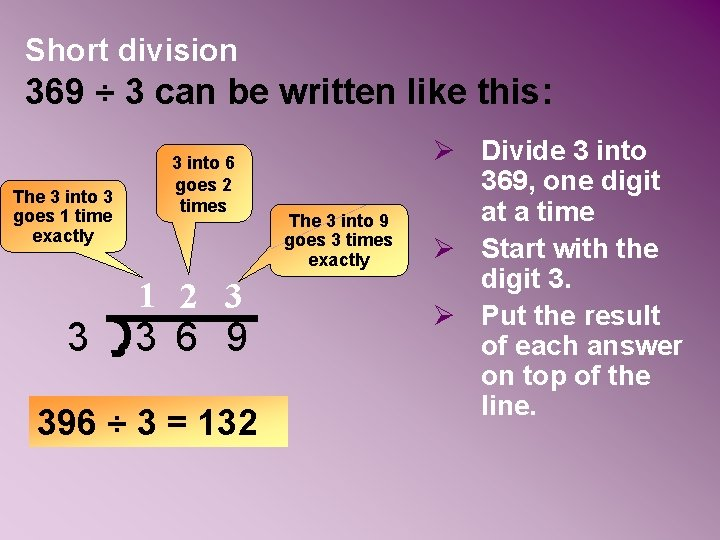Short division 369 ÷ 3 can be written like this: The 3 into 3 goes 1 time exactly 3 3 into 6 goes 2 times 1 2 3 3 6 9 396 ÷ 3 = 132 The 3 into 9 goes 3 times exactly Ø Divide 3 into 369, one digit at a time Ø Start with the digit 3. Ø Put the result of each answer on top of the line.Short division 675 ÷ 5 can be written like this: The 5 into 6 goes 1 time with 1 left over to carry 5 into 17 goes 3 times with 2 left over to carry 1 3 5 5 6 1 7 25 675 ÷ 5 = 135 The 5 into 25 goes 5 times exactly Ø Divide 5 into 675, one digit at a time Ø Start with the digit 6. Ø Put the result of each answer on top of the line.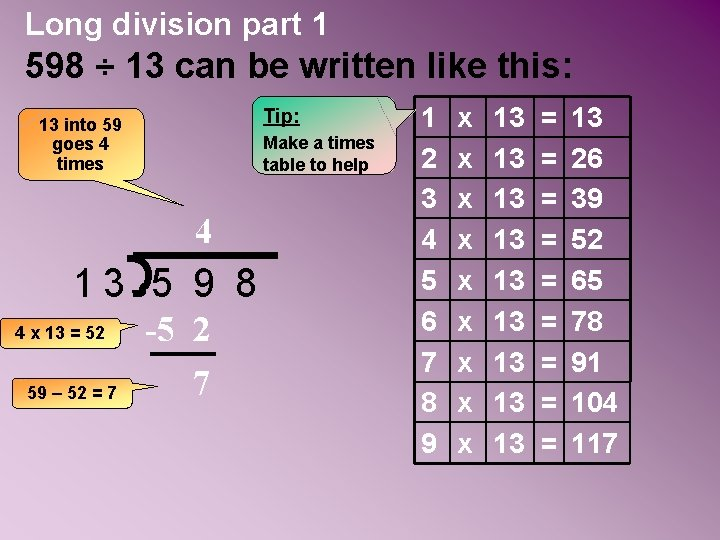Long division part 1 598 ÷ 13 can be written like this: Tip: 13 into 59 goes 4 times Make a times table to help 4 13 5 9 8 4 x 13 = 52 59 – 52 = 7 -5 2 7 1 2 3 4 5 6 7 8 9 x x x x x 13 13 13 = = = = = 13 26 39 52 65 78 91 104 117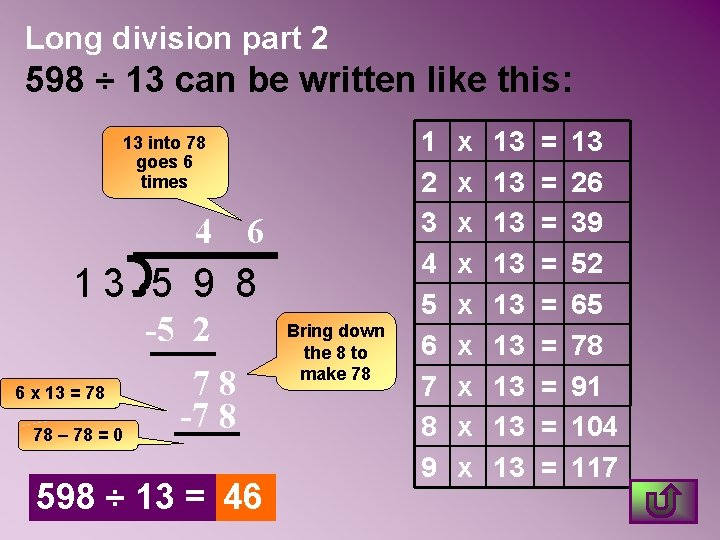Long division part 2 598 ÷ 13 can be written like this: 13 into 78 goes 6 times 4 6 13 5 9 8 6 x 13 = 78 78 – 78 = 0 -5 2 78 -7 8 598 ÷ 13 = 46 Bring down the 8 to make 78 1 2 3 4 5 6 7 8 9 x x x x x 13 13 13 = = = = = 13 26 39 52 65 78 91 104 117Long division part 1 3195 ÷ 15 can be written like this: 15 into 31 goes 2 times. 2 15 3 1 9 5 2 x 15 = 30. 31 – 30 = 1 -3 0 1 1 2 3 4 5 6 7 8 9 x x x x x 15 15 15 = = = = = Tip: Make a times table to help 15 30 45 60 75 90 105 120 135Long division part 2 3195 ÷ 15 can be written like this: 15 into 19 goes 1 times. 2 1 15 3 1 9 5 1 x 15 = 15 19 – 15 = 4 -3 0 19 -1 5 4 Bring down the 9 to make 19 1 2 3 4 5 6 7 8 9 x x x x x 15 15 15 = = = = = 15 30 45 60 75 90 105 120 135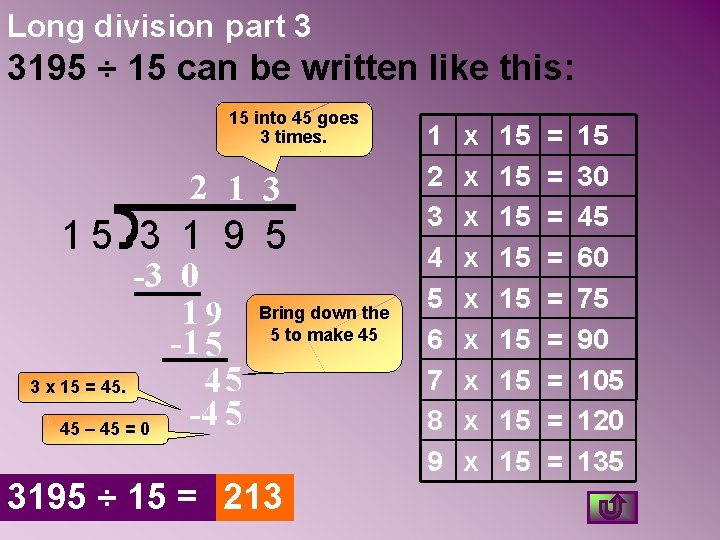Long division part 3 3195 ÷ 15 can be written like this: 15 into 45 goes 3 times. 2 1 3 15 3 1 9 5 -3 0 19 -1 5 45 3 x 15 = 45. -4 5 45 – 45 = 0 Bring down the 5 to make 45 3195 ÷ 15 = 213 1 2 3 4 5 6 7 8 9 x x x x x 15 15 15 = = = = = 15 30 45 60 75 90 105 120 135Space saver division is like short division but with larger numbers. 156 ÷ 13 can be written like this: 13 into 26 goes 2 times exactly 13 into 15 goes 1 time and 2 left over to carry 1 2 13 1 5 6 2 Ø Ø 156 ÷ 13 = 12 A times table for 13 would be helpful with this method Divide 13 into 156. Start with the digit 1, but this is not enough. Then say, 13 into 15 and continue. Put the result of each answer on top of the line.Space saver division 480 ÷ 15 can be written like this: 15 into 30 goes 2 times exactly 15 into 48 goes 3 time and 3 left over to carry 3 2 15 4 8 0 3 Ø Ø 480 ÷ 15 = 32 A times table for 15 would be helpful with this method Divide 15 into 480. Start with the digit 4, but this is not enough. Then say, 15 into 48 and continue. Put the result of each answer on top of the line.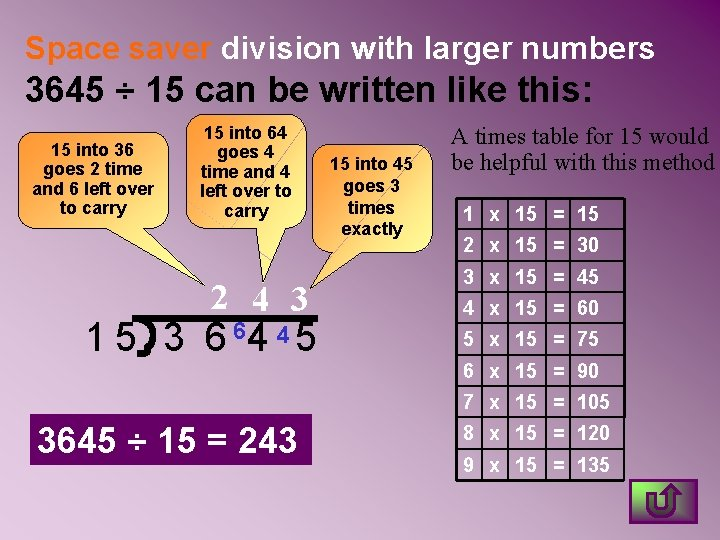Space saver division with larger numbers 3645 ÷ 15 can be written like this: 15 into 36 goes 2 time and 6 left over to carry 15 into 64 goes 4 time and 4 left over to carry 2 4 3 1 5 3 6 64 4 5 15 into 45 goes 3 times exactly A times table for 15 would be helpful with this method 1 x 15 = 15 2 x 15 = 30 3 x 15 = 45 4 x 15 = 60 5 x 15 = 75 6 x 15 = 90 7 x 15 = 105 3645 ÷ 15 = 243 8 x 15 = 120 9 x 15 = 135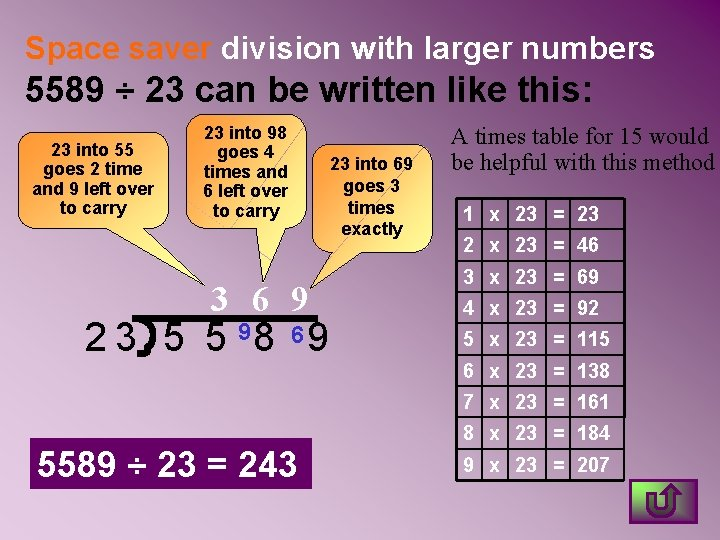Space saver division with larger numbers 5589 ÷ 23 can be written like this: 23 into 55 goes 2 time and 9 left over to carry 23 into 98 goes 4 times and 6 left over to carry 3 6 9 2 3 5 5 98 6 9 23 into 69 goes 3 times exactly A times table for 15 would be helpful with this method 1 x 23 = 23 2 x 23 = 46 3 x 23 = 69 4 x 23 = 92 5 x 23 = 115 6 x 23 = 138 7 x 23 = 161 5589 ÷ 23 = 243 8 x 23 = 184 9 x 23 = 207Space saver division with larger numbers 5535 ÷ 15 can be written like this: 15 into 55 goes 3 time and 10 left over to carry 15 into 103 goes 6 times and 13 left over to carry 3 6 9 15 into 135 goes 9 times exactly 1 5 5 5103 135 A times table for 15 would be helpful with this method 1 x 15 = 15 2 x 15 = 30 3 x 15 = 45 4 x 15 = 60 5 x 15 = 75 6 x 15 = 90 7 x 15 = 105 5535 ÷ 15 = 369 8 x 15 = 120 9 x 15 = 135Now try the exercises using both long division and space saver methods for the larger numbers